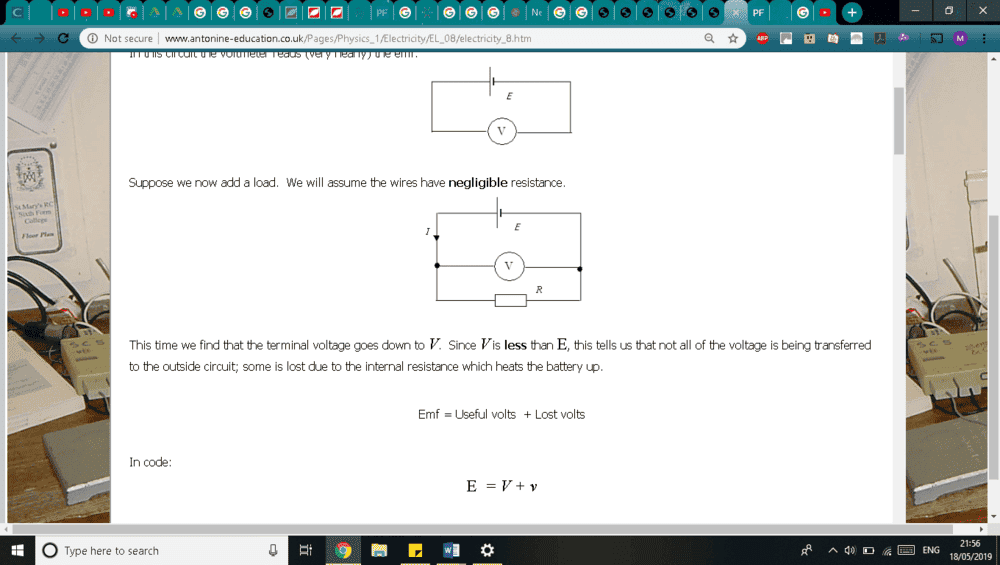# Voltmeter problem from (OCR paper) multiple choice

bonbon22
As you can see when the switch is open it measures the voltage across the battery or emf I am assuming when calculating the internal resistance when the switch is closed voltage is 6 right so the internal resistance is 6/2 =3 which is not correct. answer is A
Does this mean when the switch is ("closed" edit ) then the voltmeter now measures the terminal pd which is the voltage across the cirucuit less its internal resistance , so in this case the voltmeter measures the voltage of the resistor in series with the internal resistance , which does give me the right answer of 1.5. Why is this the case ? why does the switch change what the volt meter is measuring when closed.

Last edited:

Homework Helper
Gold Member
2022 Award
so the internal resistance is 6/2 =3
By what reasoning?
when the switch is open then the voltmeter now measures the terminal pd which is the voltage across the cirucuit less its internal resistance
It is always measuring the internal voltage of the battery less the voltage drop across the internal resistance. That voltage drop will depend on the current.
With the switch open there is no current, so no voltage drop. When the switch is closed, what is the voltage drop across the internal resistance?

•bonbon22
bonbon22
By what reasoning?

It is always measuring the internal voltage of the battery less the voltage drop across the internal resistance. That voltage drop will depend on the current.
With the switch open there is no current, so no voltage drop. When the switch is closed, what is the voltage drop across the internal resistance?
sorry made a mistake when typing
Does this mean when the switch is ("closed" edit ) then the voltmeter now measures the terminal pd which is the voltage across the cirucuit less the voltage drop on the internal resistance , so in this case the voltmeter measures the voltage of the resistor in series with the internal resistance , which does give me the right answer of 1.5. Why is this the case ? why does the switch change what the volt meter is measuring when closed.

Mentor
When the switch is closed so that current is flowing, the voltmeter is measuring the sum of the emf of the battery and the potential drop across the internal resistance.

It's also measuring the potential across the resistor R, but that's not helpful here as we don't (at this point) have a value for R.

•bonbon22
bonbon22
When the switch is closed so that current is flowing, the voltmeter is measuring the sum of the emf of the battery and the potential drop across the internal resistance.

It's also measuring the potential across the resistor R, but that's not helpful here as we don't (at this point) have a value for R.
So your telling me that when closed the voltmeter is essentially subtracting pd drop from the internal resistor from the emf which is 9 -3 = 6 <---- voltage reading ?

Homework Helper
Gold Member
2022 Award
why does the switch change what the volt meter is measuring
Look at the dashed rectangle in the diagram. The voltmeter is always measuring the voltage difference across the whole rectangle. That consists of a voltage difference across the theoretical 'ideal' battery components shown in the rectangle plus the voltage difference across the resistor. (I say "plus", not "minus", because I am assuming we assess these voltages as signed values measured in the same direction, so in the present case they will have opposite signs.)
Closing the switch changes the voltage difference across the resistor but not that across the ideal batteries, so what the voltmeter reads changes.

You may in future come on a question that involves charging a battery that has an internal resistance. In this case the current flows the other way, so the two internal voltage differences are the same sign and the voltmeter would show a higher reading when the switch is closed.

•bonbon22
bonbon22
Look at the dashed rectangle in the diagram. The voltmeter is always measuring the voltage difference across the whole rectangle. That consists of a voltage difference across the theoretical 'ideal' battery components shown in the rectangle plus the voltage difference across the resistor. (I say "plus", not "minus", because I am assuming we assess these voltages as signed values measured in the same direction, so in the present case they will have opposite signs.)
Closing the switch changes the voltage difference across the resistor but not that across the ideal batteries, so what the voltmeter reads changes.

You may in future come on a question that involves charging a battery that has an internal resistance. In this case the current flows the other way, so the two internal voltage differences are the same sign and the voltmeter would show a higher reading when the switch is closed.

haruspex is god,,, one last question would this circuit where the voltmeter is parallel to the resistor be the same as the one shown in the question? just arranged differently?sojsail
Yes, the procedures done to go between the 2 views in the 2 cases are equivalent. In the first, the external resistor is added by throwing the switch, in the second, the external resistor is added by getting out the soldering iron (or similar method of connection).